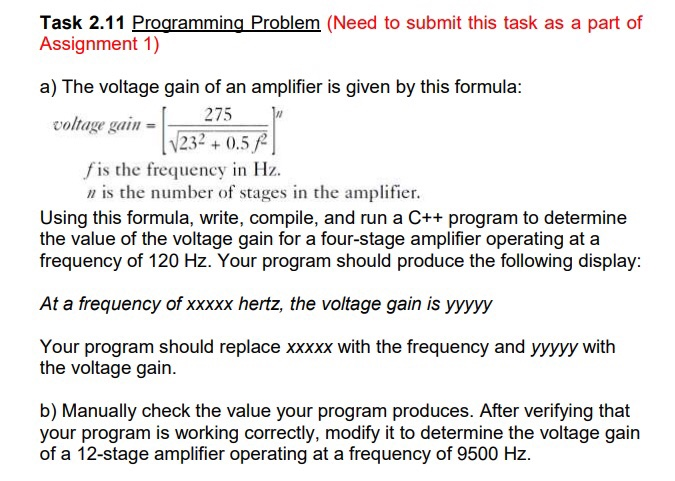Task 2.11 Programming Problem (Need to submit this task as a part of Assignment 1) a) The voltage gain of an amplifier is given by this formula: voltage gain _ 275 for (V232 +0.51) fis the frequency in Hz. n is the number of stages in the amplifier. Using this formula, write, compile, and run a C++ program to determine the value of the voltage gain for a four-stage amplifier operating at a frequency of 120 Hz. Your program should produce the following display: At a frequency of xxxxx hertz, the voltage gain is yyyyy Your program should replace XXXXX with the frequency and yyyyy with the voltage gain. b) Manually check the value your program produces. After verifying that your program is working correctly, modify it to determine the voltage gain of a 12-stage amplifier operating at a frequency of 9500 Hz.